Organic (2019 VCE) 1) The following diagram represents the structure of a non-essential vitamin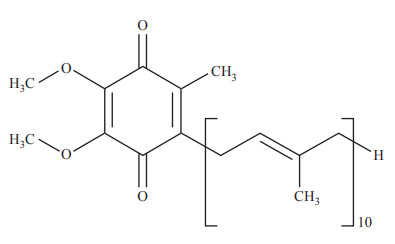This vitamin is A. fat-soluble and can be produced by the human body. B. water-soluble and can be produced by the human body. C. fat-soluble and cannot be produced by the human body. D. water-soluble and cannot be produced by the human body Solution2)Which one of the following statements about flashpoints is correct? A. The flashpoint of butane is lower than 25 °C. B. As a flashpoint increases, the viscosity decreases. C. The flashpoint of a compound is higher than its boiling point. D. The flashpoint of butane is greater than the flashpoint of butan-1-ol. Solution3) Aspartame has only A. one chiral centre. B. two stereoisomers. C. four optical isomers. D. three structural isomers. Solution4) The number of carbon-to-carbon double bonds (C=C) in a molecule can be identified by reacting the molecule with hydrogen gas, H2. The type of reaction involved is an addition reaction. 10.0 g of a fatty acid becomes fully saturated after reacting with 0.263 g of H2. The fatty acid is A. oleic acid (M = 282 g mol-1). B. linolenic acid (M = 278 g mol-1). C. arachidic acid (M = 312 g mol-1) D. arachidonic acid (M = 304 g mol-1). Solution4) Lactose is commonly found in milk and milk products. The enzyme used to hydrolyse lactose in the human small intestine is lactase. Lactose intolerance describes the limited ability of humans to hydrolyse lactose. Consider the following statements.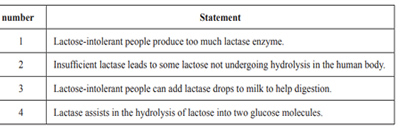Which one of the following sets of statements is correct? A. statement numbers 1, 2 and 3 B. statement numbers 1, 2 and 4 C. statement numbers 2 and 3 D. statement numbers 3 and 4 Solution5) A commercial chocolate spread is commonly used in sandwiches and desserts. This food contains high amounts of proteins, triglycerides and sucrose. Proteins are an important part of food. Proteins are broken down into smaller molecules during digestion. a. Proteins can be hydrolysed to produce alpha (α-) amino acids. Identify one structural feature common to all alpha (α-) amino acids. b. i. What is the process by which amino acids are obtained from the chocolate spread? ii. Identify the chemical process in which amino acids are predominantly used in the body. iii. Two of the amino acids in the chocolate spread are aspartic acid and cysteine. Draw the chemical structure of the dipeptide Cys-Asp at high pH Solutionc. The triglycerides in the chocolate spread contain palmitic acid and palmitoleic acid. Which of these fatty acids is likely to have a higher melting point? Explain your reasoning. Solution d. Draw the structure of a triglyceride that contains only palmitoleic acid using semi-structural formulas. Circle and label the triglyceride functional group Solution6) The following diagram represents a reaction pathway for the synthesis of Compound Q from pent-2-ene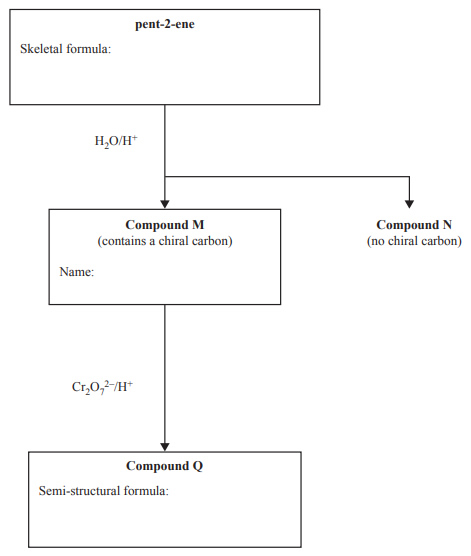i. Draw the skeletal formula for pent-2-ene in the box provided. Two structural isomers are possible when pent-2-ene is hydrolysed at a high temperature in the presence of an acid catalyst. Compounds M and N are formed. Compound M has a chiral carbon, but Compound N does not. ii. Give the IUPAC name of Compound M in the box provided. iii. When Compound M is reacted with acidified dichromate ions, Cr2O72-, Compound Q is formed. Draw the semi-structural formula of Compound Q in the box provided. Solution7) 2-chloropropane can be reacted with ammonia to produce an uncharged organic molecule, Compound R. i. Write the equation for the reaction that occurs. ii. Give the IUPAC name of Compound R. iii. Name the type of reaction that produces Compound R. iv. Calculate the percentage atom economy for the production of Compound R. Solution8) Sucrose and aspartame are types of sweeteners. Use your understanding of chemistry to explain why some people replace sugar with aspartame. In your answer, compare sucrose and aspartame in terms of: • metabolic reactions • glycaemic indices • energy content. Solution9) There are many varieties of bread available to consumers in Australia. The nutritional values for one type of wholemeal bread are given in the table below.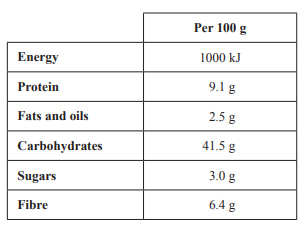a. Calculate the energy, in kilojoules, provided by the protein and fats and oils in 100 g of this wholemeal bread. b. Name a model used to explain the mechanism by which an enzyme can break down proteins. c. Proteins can be denatured by an increase in temperature. Describe what happens to the bonding in the protein structure when it is denatured by an increase in temperature. Solution d. Carbohydrates can be present in the body in many forms. i. Name the polysaccharide that is used to store energy within the body. ii. Explain the difference in the glycaemic index of amylose and amylopectin. e. Canola oil is one of the usual ingredients in bread. Canola oil is a source of omega-3 fatty acids. Why are these fatty acids classified as omega-3? Solutionf. The wholemeal bread undergoes complete combustion in a bomb calorimeter containing 200 g of water. Assume that all of the energy in the combustion is transferred to the water. i. Calculate the mass of bread needed to raise the temperature of the water by 6 °C. ii. The combustion of the bread was investigated using a different method. The bread was ignited under a beaker containing 200 g of water, which was set on a tripod. The equipment used is shown below.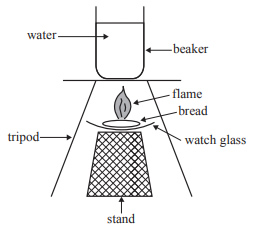If 1.2 g of bread was needed to raise the temperature of the water by 6 °C using this different method, calculate the efficiency of the energy transfer in this combustion Solution